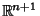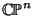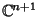## Projective Space

A Space which is invariant under the Groupof all general Linear homogeneous transformation in the Space concerned, but not under all the transformations of any Group containingas a Subgroup.

A projective space is the space of 1-D Vector Subspaces of a given Vector Space. For Real Vector Spaces, the Notationordenotes the Real projective space of dimension(i.e., the Space of 1-D Vector Subspaces of) anddenotes the Complex projective space of Complex dimension(i.e., the space of 1-D Complex Vector Subspaces of).can also be viewed as the set consisting oftogether with its Points at Infinity.